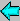## Constructing Regular Polygons -- Primitive Roots of Unity

I have been working for quite a while on finding closed, simple expressions for primitive roots of unity, that is finding solutions of cyclotomic polynomials by radical expressions in the case of constructible regular polygons.

The long-term goal is to find solutions for the 5th, 17th, 257th, and 65537th root of unity. This is an onging project.

### Primitive 5th Root of Unity$\sqrt{1} = -\frac{1}{4} \quad +$$\frac{1}{4} \cdot \sqrt{5} \quad +$$\frac{1}{2} \cdot \sqrt{-\frac{5}{2} -\frac{1}{2} \cdot \sqrt{5}}$

### Primitive 17th Root of Unity$\sqrt{1} = -\frac{1}{16} \quad +$$\frac{1}{16} \cdot \sqrt{17} \quad +$$\frac{1}{8} \cdot \sqrt{\frac{17}{2} -\frac{1}{2} \cdot \sqrt{17}} \quad +$$\frac{1}{4} \cdot \sqrt{\frac{17}{4} + \frac{3}{4} \cdot \sqrt{17} -\frac{1}{2} \cdot \sqrt{\frac{85}{2} + \frac{19}{2} \cdot \sqrt{17}}} \quad +$$\frac{1}{4} \cdot \sqrt{ -\frac{17}{2} + \frac{1}{2} \cdot \sqrt{17} - \sqrt{\frac{17}{2} -\frac{1}{2} \cdot \sqrt{17}} + \sqrt{17 + 3 \cdot \sqrt{17} + \sqrt{170 + 38 \cdot \sqrt{17}}} }$Go back